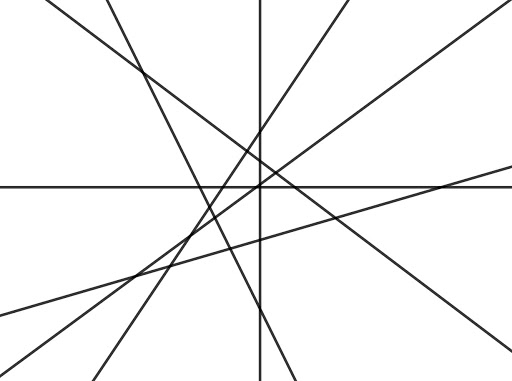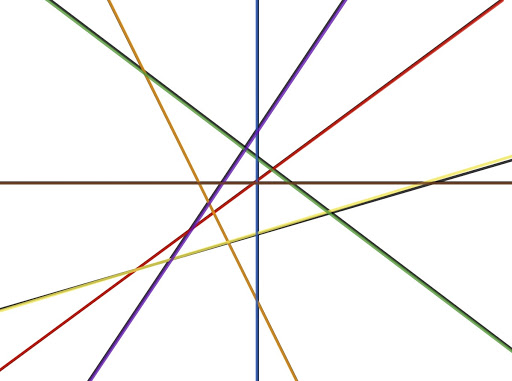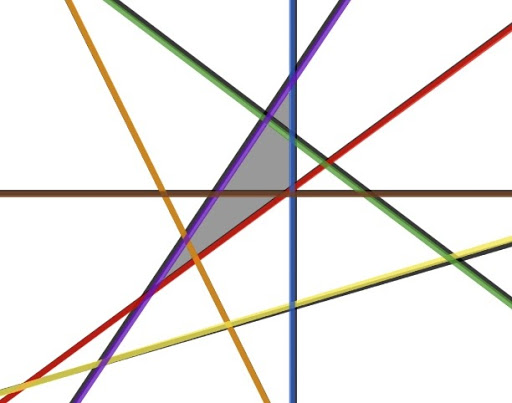# How Many triangles are in this picture: a brain teaser

### December 2nd, 2020. By Audrey Tam '22

Hint: It’s not counting them all individually. With some math, finding the answer is a lot simpler than you might think!A triangle is always made up of three lines. As you can see in the color-coded version of the picture below, there are seven total lines that intersect to form many triangles. So, out of those seven total lines, how can you choose three lines to form triangles from?To calculate the answer, we start off with how many choices we have. Since it’s established that there are seven total lines that make up the structure, we have seven choices for which colored line makes up the first “leg” of the triangle. After this, we need to pick another colored line to be our triangle’s second “leg”.

Even though it might appear that we have seven choices again, we actually have six. This is because a colored line-- let’s just say purple-- can’t be both the first and second “leg” of a triangle. So we eliminate one choice as the number of “legs” increases. When we find the third “leg” of the triangle, the same concept applies. If we chose purple and blue (or any other two colors) for our first two lines, there are five remaining lines to choose from.

After that, the math boils down to multiplying and dividing. We know that the number of total combinations of lines for triangle formation is 7x6x5, which equals 210. But there obviously aren’t 210 triangles in this picture. So what went wrong? Actually, the calculation itself is correct. But, we have also counted some combinations more than once. Luckily, finding these redundancies is not difficult.

Choosing from any three lines arbitrarily is just like choosing the order of letters A, B, and C. We can jumble them to create ABC. BCA, CAB, ACB, BAC, and CBA. Even though each sequence contains the same letters, there are six ways to shuffle these letters. Let’s say for our third line, we picked red. Mathematically, based on our calculation, our triangle could be made from lines:

1. Purple, blue, red

2. Blue, red, purple

3. Red, purple, blue

4. Purple, red, blue

5. Blue, purple, red

6. Red, blue, purpleBut these all describe the same triangle. As any triangle in the diagram can be created in six ways, we can get rid of the redundancies by dividing our number of total possibilities, 210, by 6. This leaves us with 35, which is the correct answer.

The cool thing is that this works for any number of nonparallel lines. We can use this procedure to find out that in a drawing with 35 lines, there are 6545 triangles (35x34x33 is 39270, which divided by 6 equals 6545). Or that in a picture with 7863 lines, there are 80,993,070,010 triangles-- try counting that!

This brain teaser serves as a very brief introduction to combinatorics, an area of math that works with counting and arranging. But it also illustrates the beauty of math, that it helps us identify trends which we can adapt to seemingly different situations.

TL;DR: The answer is 35, but we can use the math behind our calculation to find the number of triangles in any given number of nonparallel lines.

Appendices:

- For a broad introduction to combinatorics: https://brilliant.org/wiki/combinatorics/